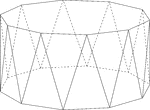Decagonal Antiprism

Illustration of a decagonal antiprism. An antiprism is formed by having two parallel congruent bases…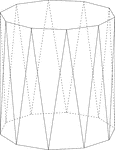Decagonal Antiprism

Illustration of a decagonal antiprism. An antiprism is formed by having two parallel congruent bases…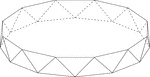Dodecagonal Antiprism

Illustration of a dodecagonal antiprism. An antiprism is formed by having two parallel congruent bases…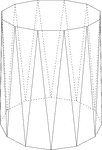Dodecagonal Antiprism

Illustration of a dodecagonal antiprism. An antiprism is formed by having two parallel congruent bases…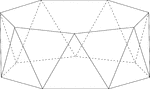Heptagonal/Septagonal Antiprism

Illustration of a heptagonal, or sometimes known as a septagonal antiprism. An antiprism is formed by…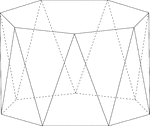Heptagonal/Septagonal Antiprism

Illustration of a heptagonal, or sometimes known as a septagonal antiprism. An antiprism is formed by…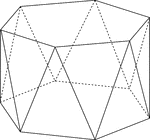Hexagonal Antiprism

Illustration of a hexagonal antiprism. An antiprism is formed by having two parallel congruent bases…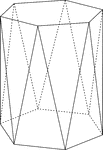Hexagonal Antiprism

Illustration of a hexagonal antiprism. An antiprism is formed by having two parallel congruent bases…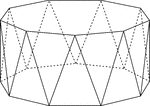Nonagonal Antiprism

Illustration of a nonagonal antiprism. An antiprism is formed by having two parallel congruent bases…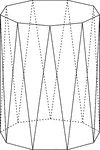Nonagonal Antiprism

Illustration of a nonagonal antiprism. An antiprism is formed by having two parallel congruent bases…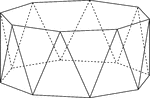Octagonal Antiprism

Illustration of an octagonal antiprism. An antiprism is formed by having two parallel congruent bases…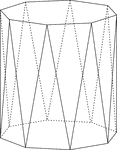Octagonal Antiprism

Illustration of an octagonal antiprism. An antiprism is formed by having two parallel congruent bases…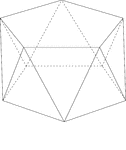Pentagonal Antiprism

Illustration of a pentagonal antiprism. An antiprism is formed by having two parallel congruent bases…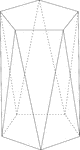Pentagonal Antiprism

Illustration of a pentagonal antiprism. An antiprism is formed by having two parallel congruent bases…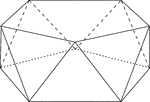Pentagonal Antiprism

Illustration of a pentagonal antiprism. An antiprism is formed by having two parallel congruent bases…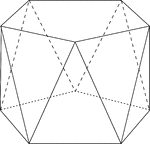Pentagonal Antiprism

Illustration of a pentagonal antiprism. An antiprism is formed by having two parallel congruent bases…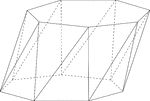Skewed Heptagonal/Septagonal Antiprism

Illustration of a skewed (non-right) heptagonal, or sometimes known as a septagonal antiprism. An antiprism…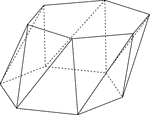Skewed Hexagonal Antiprism

Illustration of a skewed (non-right) hexagonal antiprism. An antiprism is formed by having two parallel…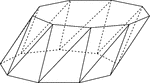Skewed Nonagonal Antiprism

Illustration of a skewed (non-right) nonagonal antiprism. An antiprism is formed by having two parallel…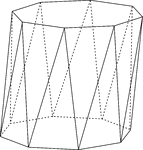Skewed Octagonal Antiprism

Illustration of a skewed (non-right) octagonal antiprism. An antiprism is formed by having two parallel…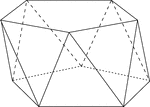Skewed Pentagonal Antiprism

Illustration of a skewed (non-right) pentagonal antiprism. An antiprism is formed by having two parallel…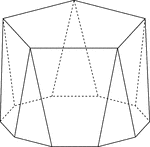Polyhedron With Pentagon Bases

Illustration of a pentagonal polyhedron that is formed by having two parallel congruent pentagonal bases…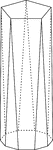Polyhedron With Pentagon Bases

Illustration of a pentagonal polyhedron that is formed by having two parallel congruent pentagonal bases…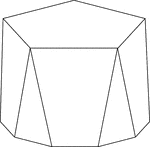Polyhedron With Pentagon Bases

Illustration of a pentagonal polyhedron that is formed by having two parallel congruent pentagonal bases…